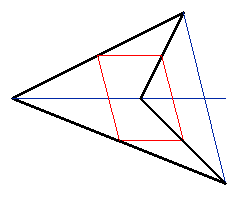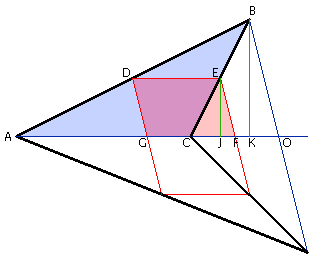In the previous section, we demonstrated that for any convex quadrilateral, the midpoint polygon would be a parallelogram with area 1/2 that of the original quadrilateral. We now consider non-convex quadrilaterals, such as the one pictured below.Figure 2. Midpoint polygon to a non-convex quadrilateral

As was the case for convex quadrilaterals, the midpoint polygon is a parallelogram with sides parallel to the diagonals of the original quadrilateral. However, we cannot use the same method to calculate the area, as one of the diagonals now lies outside the quadrilateral!

Experimentation with a simple interactive demonstration suggests that the area ration for non-convex quadrilaterals is fixed at 1/2. A slight variation on the proof given for convex quadrilaterals will establish this fact. Instead of breaking the quadrilateral into quarters, we consider two halves, divided by the diagonal that lies inside the polygon (AC in the figure below).Figure 4. Finding the area of the midpoint polygon to a non-convex quadrilateral

Because D is the midpoint of AB and E is the midpoint of BC, it follows that the length of AC is exactly twice that of DE. We then construct perpendiculars (shown in green) from E and B to AC. By similar triangles it is clear that the length of BK is twice that of EJ.

The area of triangle ABC is one-half the length of the base (AC) times the height (BK). The area of parallelogram DEFG is the length of the base (DE) times the height (EJ). But (AC)=2(DE) and (BK)=2(EJ), and so it follows that the area of the triangle ABC is twice that of the quadrilateral DEFG.

Clearly, we can carry out the same construction on the bottom half of the quadrilateral, from which it follows that the area of the midpoint polygon is half that of the original quadrilateral.